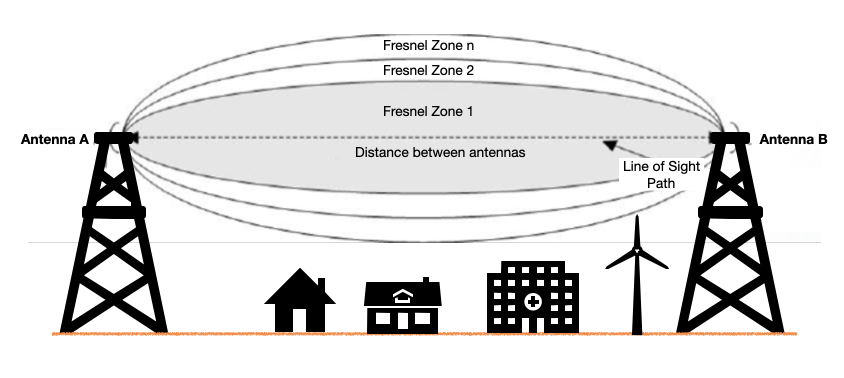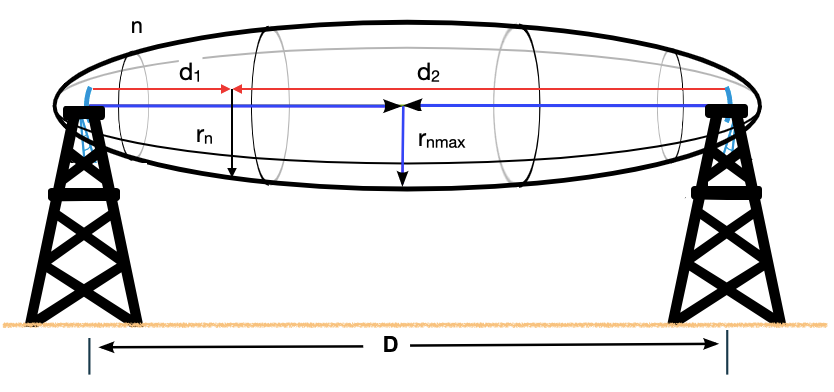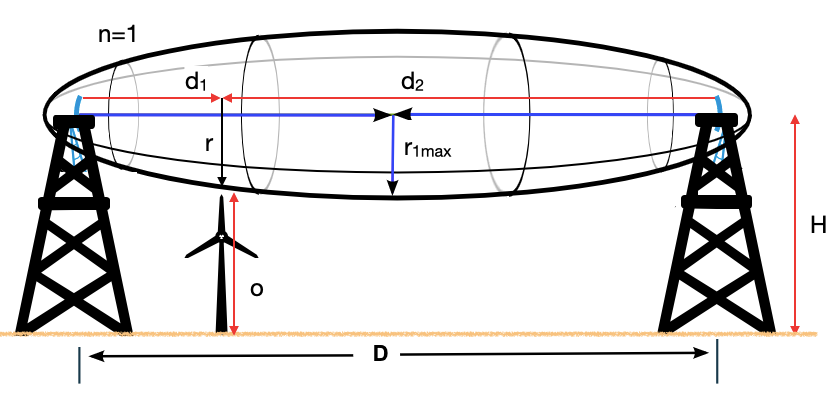# Fresnel Zone Calculator

Created by Arturo Barrantes
Reviewed by Anna Szczepanek, PhD and Steven Wooding
Last updated: Apr 06, 2022

The incredible Fresnel zone calculator allows you to determine the length of the radius at any point of the Fresnel space. Thus, you can ensure strong signal transmission between your antennas. In this article, we are going to cover everything you need to know about this topic. We'll explain in simple terms what the Fresnel zone is and provide you with the necessary formulas so that you know how to calculate Fresnel zones. We will also discuss why Fresnel zones are important and how to determine the height of your antennas to avoid signal obstruction.

## What is the Fresnel zone?

In wireless communication, we have a 3D elliptical region between the transmitter antenna and the receiver antenna. This region is determined by the distance between the antennas and the frequency of the wireless wave. It is called Fresnel Zone and looks like this:In the Fresnel zone, the longest axis of the ellipsoid is the line of sight path. Keeping it free when having constructions in the region between the antennas is critical because it can cause signal loss. However, even obstructions that do not cross the line of sight path can cause signal loss. But, how is that possible?

Wireless antennas send waves in different directions. Some waves will arrive directly at the receiver – the direct beam – and others will come after reflection with other surfaces – we call them indirect beams.

Such indirect beams travel through a longer path, meaning they get their phase angle shifted compared to the direct beam. Whenever the phase angle shifts by one-half wavelength, you get destructive interference, meaning the signals get canceled.

To avoid the harmful effects of indirect beams, we require to keep at least 60% of the 1st Fresnel zone free of obstructions. 80% is the recommended value. Subsequent Fresnel zones such as the 2nd and 3rd are also relevant but not as much as the first one. Here you can try our outstanding Fresnel Zone calculator to get an idea about the 1st zone radius and its 60% limit.

As we now understand what Fresnel zones are, let's discuss how to calculate them – in the next section we give the math formulas for Fresnel zones.

## Fresnel zone formulas

The formula for the radius of the n-th Fresnel zone reads:

rn = √(n * λ * d1 * d2 / (d1 + d2)),

where:

• λ is the wavelength of the wireless beam transmitted by the antenna; and
• d1, d2, rn as in the picture below:If we want to get the longest Fresnel zone radius (rnmax), we have to recall that an ellipsoide is the biggest at its center. That is, when we have d1 = d2 = D / 2, and the above formula simplifies to:

 rnmax = √(n * λ * D / 4)

And that's how we calculate the Fresnel zone! As you can see, it's not at all hard! To avoid any doubts, let's discuss the formulas for 1st Fresnel zone.

#### First Fresnel zone formulas

In particular, if we want to determine the radius at any point in the first Fresnel zone, we would use the following formula:

r = √(λ * d1 * d2/ (d1 + d2)), accordingly to the picture:And for the 1st Fresnel zone longest radius we have:

r1max = √(λ * D / 4)

Now, if we use the wavelength formula, we get:

 r1max = √(c * D / (4 * f)),

where f represents wireless beam frequency.

Recall that wireless waves travel at the speed of light (they are electromagnetic waves); thus, c = 300,000 km/s. Furthermore, if we input in the equation D and f in kilometers and in GHz, respectively, then we get:

 r1max = 17.32 * √(D / (4 * f)), expressed in meters.

Another way of expressing this equation is:

 r1max = 8.66 * √(D / f), expressed in meters.

## Why is the Fresnel Zone important?

As mentioned above, we have to keep 60% of the first Fresnel zone free of interference so the signal can reach the receiver antenna without considerable problems. This issue is of current concern because buildings and other structures often get in the way of the antennas and break this rule.

Let's consider wind turbines which are certainly revolutionizing the way we generate energy. Such machines can be 80 meters tall; then, the antenna height should be as tall as 80 meters plus the 1st Fresnel zone radius for getting 100% of no interference.

That is why in our fantastic Fresnel zone calculator, we have included a feature where you can add the height of the obstruction (o) and the height of the antennas (H). Please refer to our latest drawing for further reference.

## How to calculate the Fresnel zone? Examples

For the first and longest Fresnel zone, we need the distance between antennas and the frequency. Suppose that:

D = 2 km and

 f = 2.437 GHz.

Then by using our cool Fresnel zone calculator or the formula explained above, we determine the longest radius of the 1st Fresnel zone:

 r1max = 8.66 * √(2 / 2.437),

and so:

 r1max = 7.85 m.

In case we are interested in the longest radius of the 2nd Fresnel zone for the same input, we have:

 r2max = √(2 * λ * 2 / 4), where
λ = c / f.

Consequently,

 r2max = 8.66 * √(2 * 2 / 2.437),

and so:

 r2max = 11.09 m.

## How to use our Fresnel zone calculator for antenna height selection?

In the first Fresnel zone section, you have to define the place of your obstruction so you can get the Fresnel zone at that point. Assume that:

d1 = 0.6 km, d2 = 1.4 km.

Then, we get the radius of the first Fresnel zone in that point:

r = √(λ * d1 * d2 / (d1 + d2))

r = √(c * 0.6 km * 1.4 km / (2 km * 2.437 Ghz))

r = 7.19 m.

Secondly, we have to add the size of our obstruction at the desired point. Let's consider a building of 9 meters:

o = 9 m

Then, our amazing Fresnel zone calculator indicates that the antennas' height should be at least:

H = 13.31 m

We can verify it by finding the distance from the ground to the 60% Fresnel zone considering the suggested antenna height:

H - 0.6 * r = 13.31 - 0.6 * 7.19 = 9 m,

which is the maximum allowed height for any obstruction.

Finally, we invite you to check our full set of .

## FAQ

### How do I calculate the first Fresnel zone?

To calculate the radius of the first Fresnel zone, follow these steps:

1. Get the distance D between the transmitter antenna and the receiver antenna. Define it in kilometers.
2. Select the frequency f that you are going to use for wireless communication. Express it in gigaHertz (GHz).
3. Compute the square root of D / f.
4. Finally, multiply the result of the last operation by 8.66. The result is the longest radius of the 1st Fresnel zone, expressed in meters. And that's how we calculate Fresnel zones :-)

### How much of the 1st Fresnel zone shall be free?

We recommend you limit your design in a way that will leave 80% of the 1st Fresnel zone free. However, 60% is still acceptable. This precaution is because the reflected waves get their phase angle shifted. Reflections will cause signal loss or, in the worst cases, signal cancellation.

### How do I calculate the limit height of the obstruction?

Here are the steps you should follow to calculate the limit height of the obstruction:

1. First, you have to get the radius of the first Fresnel zone at the point to be checked: r.
2. You also need to know the antenna's height: H.
3. Subtract 60% of the Fresnel zone radius from the antenna's height: H - 0.6 * r.
4. The result is the maximum obstruction height you can get at the selected point of the 1st Fresnel zone.

### Can earth curvature be an obstruction?

After 5 km of distance between antennas, Earth's curvature does affect the antenna's height and, consequently, the Fresnel zone. Thus, we have to adjust the antenna's height by the following factor: Distance between antennas to the second power times 1000 divided by 8 times the Earth radius. Such a factor has to be added to the antenna's height to compensate for Earth's curvature.

### What is the shape of a Fresnel zone?

In wireless communication, we have a 3D elliptical region between the transmitter antenna and the receiver antenna. This region is determined by the distance between the antennas and the frequency of the wireless wave. It is called Fresnel Zone.

Arturo Barrantes
I want to calculate the
1st Fresnel zoneFrequency
GHz
Distance between the antennas (D)
km
m
Radius of Fresnel zone at a specific point
Distance from the emitter antenna (d₁)
km
Distance from the receiver antenna (d₂)
km
Radius of 1st Fresnel zone (r)
m
Obstruction at a specific point?
Antennas height (H)
m
Obstruction height (o)
m
People also viewed…

### Books vs e-Books

The books vs. e-books calculator answers the question: how ecological is your e-book reader? 📚

### Pizza size

This calculator will help you make the most delicious choice when ordering pizza.

### Sled

Find out if a slope is safe to sled on with our sled calcultor!

### Thermal stress

Determine the stress on an object due to heating or cooling using the thermal stress calculator.